# RD Sharma Solutions Class 9 Chapter 9 Triangle and its Angles

Read RD Sharma Solutions Class 9 Chapter 9 Triangle and its Angles below, students should study RD Sharma class 9 Mathematics available on Studiestoday.com with solved questions and answers. These chapter wise answers for class 9 Mathematics have been prepared by teacher of Grade 9. These RD Sharma class 9 Solutions have been designed as per the latest NCERT syllabus for class 9 and if practiced thoroughly can help you to score good marks in standard 9 Mathematics class tests and examinations

Exercise 9.1

Question 1: In a ΔABC, if ∠A = 55°, ∠B = 40°, find ∠C.
Solution:
Given that, ∠A = 55° and ∠B = 40°
∠A + ∠B + ∠C = 180° (angle sum property)
Put the value of ∠A and ∠B in above equation.
55° + 40° + ∠C = 180°
95° + ∠C = 180°
∠C = 180° − 95°
∠C = 85°
Thus, the value of ∠C is 85°

Question 2: If the angles of a triangle are in the ratio 1:2:3, determine three angles.
Solution:
Let the angles be x,2x,3x
Angle sum property says that all angles of triangle is 180°.
x+2x+3x=180°
6x=180°
x=(180°)/6
x=30°
x=30°
2x=2(30)° =60°
3x= 3(30)° =90°
Hence, the values of angles are 30°, 60° and 90°.

Question 3: The angles of a triangle are (x − 40)°, (x − 20)° and (1/2 x-10°). Find the value of x.
Solution:
It is given that, angles of a triangle are(x-40)°, (x-20)° and (1/2 x-10)°
Angle sum property says that all angles of triangle is 180°.
(x-40)°+(x-20)°+(1/2 x-10)°= 180°
2x+1/2 x-40°-20°-10°=180°
5/2 x – 70° = 180°
5/2 x = 180° + 70°
5x = 2(250)°
x = (500°)/5
x = 100°
Hence, the value of x is 100°.

Question 4: The angles of a triangle are arranged in ascending order of magnitude. If the difference between two consecutive angles is 10°, find the three angles.
Solution:
It is given that the difference between two consecutive angles is 10°.
Let the first angle will be x,
Second angle will be x + 10°
Third angle will be x + 20°
Angle sum property says that all angles of triangle is 180°.
x + (x + 10°) + (x + 20°) = 180°
x + x + 10°+ x + 20° = 180°
3x + 30° = 180°
3x = 180°– 30°
3x = 150°
x = (150°)/3
x = 50°
x + 10° = 50° + 10° = 60°
x + 20° = 50° + 20° = 70°
Hence, the value of angles are 50°, 60° and 70°.

Question 5: Two angles of a triangle are equal and the third angle is greater than each of those angles by 30°. Determine all the angles of the triangle.
Solution:
Two angles of a triangle are equal and the third angle is greater than each of those angles by 30°.
Let the first angle and second angle will be x
Third angle will be x + 30°
Angle sum property says that all angles of triangle is 180°.
x + x + (x + 30°) = 180°
x + x + x + 30° = 180°
3x + 30° = 180°
3x = 150°
3x = (150°)/3
x = 50°
First and second angle x = 50°
Third angle = x + 30°
Third angle = 50° + 30°
Third angle = 80°
Hence, the three angles of a triangle are 50°, 50° and 80°.

Question 6: If one angle of a triangle is equal to the sum of the other two, show that the triangle is a right angle triangle.
Solution:
It is given that one angle of a triangle is equal to the sum of the other two angles.
Let x, y and z are three angles of a triangle.
z = x + y_______(1)
Angle sum property says that all angles of triangle is 180°.
x + y + z = 180°
z + z = 180°
2z = 180°
z = 90°
One angle of a triangle is 90° hence, it is right triangle.

Question 7: ABC is a triangle in which ∠A = 72°, the internal bisectors of angles B and C meet in O. Find the magnitude of ∠BOC.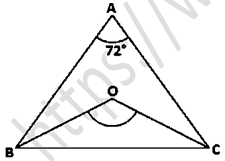Solution:
It is given that, ABC is a triangle where ∠A = 72°
In ΔABC,
Angle sum property says that all angles of triangle is 180°.
∠A + ∠B + ∠C = 180°
72° + ∠B + ∠C = 180°
∠B + ∠C = 180° − 72°
Line OB is the bisector of ∠B and Line OC is bisector of ∠C.
Dividing both sides by '2'
(∠B)/2 + (∠C)/2 = (180°)/2
∠OBC + ∠OCB = 54°
In ΔBOC
Angle sum property says that all angles of triangle is 180°.
∠OBC + ∠OCB + ∠BOC = 180°
54° + ∠BOC = 180°
∠BOC = 180° − 54°
∠BOC = 126°
Hence, the value of ∠BOC is 126°.

Question 8: The bisectors of base angles of a triangle cannot enclose a right angle in any case.
Solution: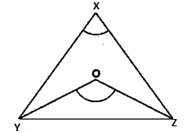It is given that ∆XYZ in which YO and CO are the bisectors of the base angles ∠B and ∠C respectively.
Let us assumed that ∠YOZ = 90°
∠ZYO + ∠YZO = 180°
1/2∠Y + 1/2∠Z = 1/2×180°
∠Y + ∠C = 180°
∠A = 0° (as per triangle sum property)
This shows that the points A, B and C do not form a triangle, which is false. So our assumption is wrong.
Hence, ∠YOZ is not a right triangle.

Question: 9 If the bisectors of the base angles of a triangle enclose an angle of 135°, prove that the triangle is a right angle.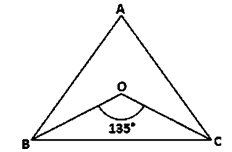Solution:
It is given that the bisectors of the base angles of a triangle enclose an angle of 135°
∠BOC = 135° (bisectors of the base angles)
But, We know that
∠BOC = 90° + 1/2∠A
135° = 90° + 1/2∠A
135° - 90° = 1/2∠A
45° = 1/2∠A
45° × 2 = ∠A
∠A = 90°
Hence, ΔABC is a right angle triangle that is right angled at A.

Question 10: In a ΔABC, ∠ABC = ∠ACB and the bisectors of ∠ABC and ∠ACB intersect at O such that ∠BOC = 120°. Show that ∠A = ∠B = ∠C = 60°.
Solution:It is given that,
∠ABC = ∠ACB
Bisectors in ΔABC,
1/2∠ABC = 1/2∠ACB
OB, OC bisects ∠B and ∠C
∠OBC = ∠OCB
∠BOC = 90° + 1/2∠A
120° = 90°+ 1/2∠A
120° - 90° = 1/2∠A
30° × 2 = ∠A
∠A = 60°
In ΔABC
Sum of all angles of a triangle is 180°.
∠A + ∠ABC + ∠ACB = 180°
60° + 2∠ABC = 180° (∠ABC = ∠ACB)
2∠ABC = 180° − 60°
∠ABC = (120°)/2
∠ABC = 60°
∠ABC = ∠ACB
∠ACB = 60°
Hence it is proved that ∠ACB is 60°.

Question 11:  Can a triangle have:-
(i) Two right angles?
(ii) Two obtuse angles?
(iii) Two acute angles?
(iv) All angles more than 60°?
(v) All angles less than 60°?
(vi) All angles equal to 60°?
Solution:
(i) No
(ii) No
(iii) Yes
(iv) No
(v) No
(vi) Yes

Question 12: If each angle of a triangle is less than the sum of the other two, show that the triangle is acute angled.
Solution:It is given that, each angle of a triangle less than the sum of the other two
∠A < ∠B + ∠C
∠B < ∠C + ∠A
∠C < ∠A + ∠B
∠A + ∠B + ∠C = 180° (Angle sum property)
∠A < ∠B + ∠C
∠A +∠A < ∠A + ∠B + ∠C
2∠A < 180°
∠A < 90°
Similarly,
∠B < 90°
∠C < 90°
Hence, it is proved that the triangles are acute angle.

Exercise 9.2

Question 1: The exterior angles, obtained on producing the base of a triangle both ways are 104° and 136°. Find all the angles of the triangle.
Solution: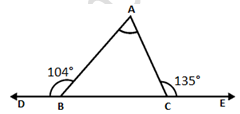It is given that, DBC is a line.
∠DBA + ∠ABC = 180°
104° + ∠ABC = 180°
∠ABC = 180° - 104°
∠ABC = 76°
∠ACE + ∠ACB = 180°
135° + ∠ACB = 180°
∠ACB = 180° - 135°
∠ABC = 45°
By the angle sum property
∠BAC + ∠ABC + ∠ACB = 180°
∠BAC + 76° + 45° = 180°
∠BAC + 121° = 180°
∠BAC = 180° - 121°
∠BAC = 59°
Hence, the value of angles of a triangle are ∠A = 59°, ∠B = 76° and ∠C = 45°.

Question 2: In a triangle ABC, the internal bisectors of ∠B and ∠C meet at P and the external bisectors of ∠B and ∠C meet at Q. Prove that ∠BPC + ∠BQC = 180°.
Solution: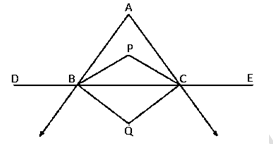Let us assumed that,
∠ABD = 2x and ∠ACE = 2y
∠ABC = 180° − 2x (linear pair)
∠ACB = 180° − 2y (linear pair)
∠A + ∠ABC + ∠ACB = 180° [Sum of all angles of a triangle]
∠A + 180° − 2x + 180° − 2y = 180°
−∠A + 2x + 2y = 180°
x + y = 90° + 1/2∠A
Now in ΔBQC
x + y + ∠BQC = 180° [Sum of all angles of a triangle]
90° + 1/2∠A + ∠BQC = 180°
∠BQC = 90° - 1/2∠A_____(1)
Adding (i) and (ii) we get ∠BPC + ∠BQC = 180°
Hence proved.

Question 3: In figure, the sides BC, CA and AB of a △ABC have been produced to D, E and F respectively. If ∠ACD = 105° and ∠EAF = 45°, find all the angles of the △ABC.Solution:
It is given that,
∠EAF = 45°
∠BAC = ∠EAF (Vertically opposite angles)
∠BAC = 45°
∠ACB + ∠ACD = 180° (Linear pair)
∠ACB + 105° = 180°
∠ACD = 180° – 105°
∠ACD = 75°
By the angle sum property, we get
∠BAC + ∠ACB + ∠ABC = 180°
45° + 75° + ∠ABC = 180°
105° + ∠ABC = 180°
∠ABC = 105° – 45°
∠ABC = 60°
Hence the value of ∠ABC = 60°, ∠ACD = 75° and ∠BAC = 45°.

Question 4: Compute the value of x in each of the following figures:
(i)Solution:
EAC is a line,
∠EAB + ∠BAC = 180° (Linear Pair)
120° + ∠BAC = 180°
∠BAC = 180° - 120°
∠BAC = 60°
BCD is also a line
∠ACB + ∠ACD = 180° (Linear Pair)
∠ACB + 112° = 180°
∠ACB = 180° - 112°
∠ACB = 180° - 112°
∠ACB = 68°
Sum of all angles of a triangle is 180°
Let ∠ABC is x
∠ABC + ∠BAC + ∠ACB = 180°
x + 60° + 68° = 180°
x + 128° = 180°
x=180°-128°
x=52°
Hence, the value of x is 52°Solution:
It is given that, EBCD lies on the same base so it is a line.
∠ABE + ∠ABC = 180° (Linear pair)
120° + ∠ABC = 180°
∠ABC = 180° - 120°
∠ABC = 60°
∠ACD + ∠ACB = 180° (Linear pair)
110° + ∠ACB = 180°
∠ACB = 180° - 110°
∠ACB = 70°
Sum of all angles of a triangle is 180°
∠ABC + ∠ACB + ∠BAC = 180°
60° + 70° + ∠BAC = 180°
130° + ∠BAC = 180°

∠BAC = 180° − 130°
∠BAC = 50°
∠BAC = x
x = 50°
Hence, the value of x is 50°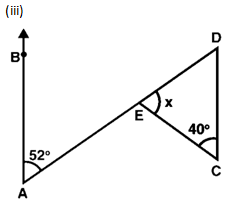Solution:
∠BAE = ∠EDC = 52° (Alternate angles)
Sum of all angles of a triangle is 180°.
∠ECD + ∠EDC + ∠CED = 180°
40° + 52° + ∠CED = 180°
92° + ∠CED = 180°
∠CED = 180° - 92°
∠CED = 88°
∠CED = x
x = 88°
Hence, the value of angle x is 88°.Solution:
In triangle ∆ABE,
∠BAE + ∠ABE + ∠BEA = 180° (angle sum property)
35° + 45° + ∠BEA = 180°
80° + ∠BEC = 180°
∠BEC = 180° - 80°
∠BEC = 100°
∠BED + ∠DEC = 180°
100° + ∠DEC = 180°
∠DEC = 180° - 100°
∠DEC = 80°
By exterior angle property
∠CDA = ∠DEC + ∠DCE
∠CDA = 80° + 50°
∠CDA = 130°
Hence, the value of angle ∠CDA is 130°.

Question 5: In figure, AB divides ∠DAC in the ratio 1 : 3 and AB = DB. Determine the value of x.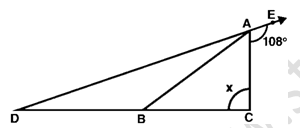Solution:
It is given that in figure,
∠EAC = 108°
∠DAC + ∠EAC = 180°
∠DAC + 108° = 180°
∠DAC = 180° − 108°
∠DAC = 72°
(∠BAC)/(∠DAB) = 1/3
3∠BAC = ∠DAB
∠DAC = ∠BAC + ∠DAB
72° = ∠BAC + ∠DAB
Put the value of ∠DAB as 3∠BAC.
∠BAC + 3∠BAC = 72°
4∠BAC = 72°
∠BAC = 72/4
∠BAC = 18°
So we get
∠DAB = 3 × 18°
∠DAB = 54°
∠DAB = ∠BDA = 54° (as AB = DB)
∠ABD = 180° − (54° + 54°)
∠ABD = 180 − 108°
∠ABD = 72°
Now,
∠DBA = 72° = ∠BAC + x (Exterior angle)
So we get
x =72°−18°
x = 54°
Hence, the value of angle x is 54°.

Question 6: ABC is a triangle. The bisector of the exterior angle at B and the bisector of ∠C intersect each other at D. Prove that ∠D = 1/2∠A.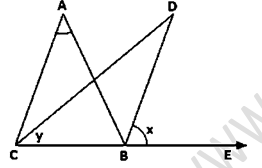Solution:
Let ∠ABE = 2x and ∠ACB = 2y
ABC is a triangle ∠ABC = 180° − 2x [Linear pair]
∠A = 180° − ∠ABC − ∠ACB [Angle sum property]
= 180° − 180° + 2x + 2y
= 2(x − y) ..... (i)
Now, ∠D = 180° − ∠DBC − ∠DCB
∠D = 180° − (x + 180° − 2x) − y
∠D = 180° − x − 180° + 2x − y
(x − y)

Question 7: In figure 9.36, AC ⊥ CE and ∠A:∠B: ∠C = 3: 2: 1, findSolution:
It is given that,
∠A : ∠B : ∠C = 3 : 2 : 1
Let us assumed that the angles be 3x, 2x and x
∠A + ∠B + ∠C = 180° (Angle sum property)
3x + 2x + x = 180°
6x = 180°
x = 30°
∠ACB = x = 30°
∠ABC = 2x = 2 × 30°
∠ABC = 60°
From the figure we can that,
∠ACE = 90°
BCD is a line
∠BCA + ∠ACE + ∠ECD = 180°
30° + 90° + ∠ECD = 180°
120° + ∠ECD = 180°
∠ECD = 180°- 120°
∠ECD = 60°
Hence, the value of ∠ ECD is 60°.

Question 8: In figure 9.37, AM ⊥ BC and AN is the bisector of ∠A. If ∠B = 65° and ∠C = 33°, find ∠MAN.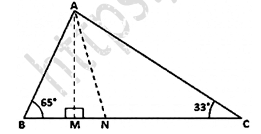Solution:
Let us assumed that,
∠BAN = ∠NAC = x (AN bisects ∠A)
∠ANM = x + 33° (Exterior angle property)
In ΔAMB
∠BAM = 90° − 65° (Exterior angle property)
∠BAM = 25°
∠MAN = ∠BAN − ∠BAM
∠MAN = x − 25°
In ΔMAN,
∠MAN + ∠ANM + ∠AMN = 180° (Angle sum property)
(x - 25)° + (x + 33)° + 90° = 180°
x - 25° + x + 33° + 90° = 180°
2x + 8° + 90° = 180°
2x + 98° = 180°
2x = 180° - 98°
2x = 82°
x = (82°)/2
x = 41°
∠MAN = x − 25°
∠MAN = 41° − 25°
∠MAN = 16°
Hence, the value of ∠MAN is 16°.

Question 9: In a triangle ABC, AD bisects ∠A and ∠C > ∠B. Prove that ∠ADB > ∠ADC.Solution:
It is given that,
∠C > ∠B __________(1)
∠A + ∠C + ∠ ADC = 180° (Linear Pair)
x + ∠C + ∠ ADC = 180°
x + ∠C = 180° - ∠ ADC
∠A + ∠B + ∠ ADB = 180° (Linear Pair)
x + ∠B + ∠ ADB = 180°
x + ∠B = 180° - ∠ ADB
Adding x on both sides of equation 1.
∠C + x > ∠B + x
Hence proved

Question 10:  In triangle ABC, BD ⊥ AC and CE ⊥ AB. If BD and CE intersect at O, prove that ∠BOC = 180° - ∠A.Solution:
From the figure we know that, AEOD is a quadrilateral.
∠A + ∠AEO + ∠EOD + ∠ADO = 360° (Sum of all four angle of a quadrilateral is 360°)
∠A + 90° + 90° + ∠EOD = 360°
∠A + 180° + ∠EOD = 360°
∠A + ∠EOD = 360° - 180°
∠A + ∠EOD = 180°
∠EOD = ∠BOC (vertically opposite angles)
∠A + ∠BOC = 180°
∠BOC = 180° − ∠A
Hence Proved

Question 11:  In figure 9.38, AE bisects ∠CAD and ∠B = ∠C. Prove that AE ∥ BC.Solution:
Let us assumed that ∠B = ∠C = x
We know that,
∠CAD = 2x (exterior angle is sum of interior angles)
∠EAC = x
∠EAC = ∠C
Hence it is proved that, these are the alternate interior angles for the lines AE and BC. AE ∥ BC

Question 12:  In figure 9.39, AB ∥ DE. Find ∠ACD.Solution:
It is given that, AB ∥ DE
∠ABC = ∠CDE = 40° (Alternate angles)
∠ABC + ∠ACB + ∠BAC = 180° (Angle sum property)
40° + ∠ACB + 30° = 180°
∠ACB = 180° − 40° − 30°
∠ACB = 110°
∠ACD = 180° − 110° (Linear pair)
∠ACD = 70°
Hence, the value of ∠ACD is 70°.

Question 13;  Which of the following statements are true (T) and which are false (F):
(i) Sum of the three angles of a triangle is 180°.
(ii) A triangle can have two right angles.
(iii) All the angles of a triangle can be less than 60°.
(iv) All the angles of a triangle can be greater than 60°.
(v) All the angles of a triangle can be equal to 60°.
(vi) A triangle can have two obtuse angles.
(vii) A triangle can have at most one obtuse angles.
(viii) If one angle of a triangle is obtuse, then it cannot be a right angled triangle.
(ix) An exterior angle of a triangle is less than either of its interior opposite angles.
(x) An exterior angle of a triangle is equal to the sum of the two interior opposite angles.
(xi) An exterior angle of a triangle is greater than the opposite interior angles.
Solution:
(i) T
(ii) F
(iii) F
(iv) F
(v) T
(vi) F
(vii) T
(viii) T
(ix) F
(x) T
(xi) T

Question 14 : Fill in the blanks to make the following statements true:
(i) Sum of the angles of a triangle is _______ .
(ii) An exterior angle of a triangle is equal to the two ________ opposite angles.
(iii) An exterior angle of a triangle is always ________ than either of the interior opposite angles.
(iv) A triangle cannot have more than _______ right angles.
(v) A triangles cannot have more than _______ obtuse angles.
Solution:
(i) 180°
(ii) Interior
(iii) Greater
(iv) One
(v) One

Exercise VSAQs ........................

Question 1: Define a triangle.
Solution:
A triangle is a three-sided polygon with three vertices and three sides. The number of a triangle's internal angles equals 180 degrees is the most essential property of a triangle.

Question 2: Write the sum of the angles of an obtuse triangle.
Solution:
180° is the sum of angles of obtuse triangle.

Question 3: In △ABC, if ∠B = 60°, ∠C = 800 and the bisectors of angles ∠ABC and ∠ACB meet at point O, then find the measure of ∠BOC.
Solution:
It is given that,
∠B = 60°, ∠C = 80°
As per question:
∠OBC = (60°)/2
∠OBC = 30°
∠OCB = (80°)/2
∠OCB = 40°
In ∆BOC,
We know that, Sum of angles of a triangle is 180°
∠OBC + ∠OCB + ∠BOC = 180° (angle sum property)
30° + 40° + ∠BOC = 180°
70° + ∠BOC = 180°
∠BOC = 180° - 70°
∠BOC = 110°
Hence, the value of ∠BOC is 110°

Question 4: If the angles of a triangle are in the ratio 2:1:3, then find the measure of smallest angle.
Solution:
Let us assumed that,
Angles of a triangles are 2x, x and 3x, where x is the smallest angle.
We know that, Sum of angles of a triangle is 180°
2x + x + 3x = 180° (angle sum property)
6x = 180°
x = 30°.
Hence, the value of angle smallest angle is 30°.

Question 5: If the angles A, B and C of △ABC satisfy the relation B – A = C – B, then find the measure of ∠B.
Solution:
We know that, Sum of angles of a triangle is 180°
∠A + ∠B + ∠C = 180°_____(1) (Angle sum property)
It is given that,
∠B – ∠A = ∠C – ∠B
2∠B = ∠C + ∠A ______(2)
Put the value of C + A in equation (1)
2∠B + ∠B = 180°
3∠B =180°
3∠B =180°
∠B = 60°
Hence, the value of ∠B is 60°.